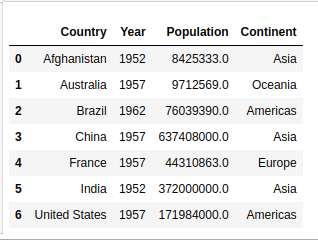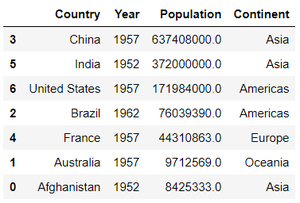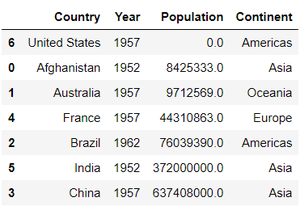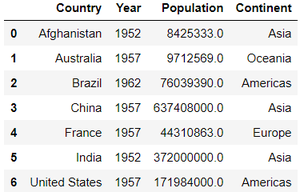# How to Sort Pandas DataFrame?

In this article, we will discuss how to sort Pandas Dataframe. Let’s create a dataframe.

Example :

## Python3

 `# importing pandas library ` `import` `pandas as pd ` ` `  `# creating and initializing a nested list ` `age_list ``=` `[[``'Afghanistan'``, ``1952``, ``8425333``, ``'Asia'``], ` `            ``[``'Australia'``, ``1957``, ``9712569``, ``'Oceania'``], ` `            ``[``'Brazil'``, ``1962``, ``76039390``, ``'Americas'``], ` `            ``[``'China'``, ``1957``, ``637408000``, ``'Asia'``], ` `            ``[``'France'``, ``1957``, ``44310863``, ``'Europe'``], ` `            ``[``'India'``, ``1952``, ``3.72e``+``08``, ``'Asia'``], ` `            ``[``'United States'``, ``1957``, ``171984000``, ``'Americas'``]] ` ` `  `# creating a pandas dataframe ` `df ``=` `pd.DataFrame(age_list, columns``=``[``'Country'``, ``'Year'``, ` `                                     ``'Population'``, ``'Continent'``]) ` ` `  `df `

Output :## Sorting Pandas Data Frame

In order to sort the data frame in pandas, function sort_values() is used. Pandas sort_values() can sort the data frame in Ascending or Descending order.

Example 1: Sorting the Data frame in Ascending order

## Python3

 `# importing pandas library ` `import` `pandas as pd ` ` `  `# creating and initializing a nested list  ` `age_list ``=` `[[``'Afghanistan'``,``1952``,``8425333``,``'Asia'``], ` `            ``[``'Australia'``,``1957``,``9712569``,``'Oceania'``], ` `            ``[``'Brazil'``,``1962``,``76039390``,``'Americas'``], ` `            ``[``'China'``,``1957``,``637408000``,``'Asia'``], ` `            ``[``'France'``,``1957``,``44310863``,``'Europe'``], ` `            ``[``'India'``,``1952``,``3.72e``+``08``,``'Asia'``], ` `            ``[``'United States'``,``1957``,``171984000``,``'Americas'``]] ` ` `  `# creating a pandas dataframe ` `df ``=` `pd.DataFrame(age_list,columns``=``[``'Country'``,``'Year'``, ` `                                    ``'Population'``,``'Continent'``]) ` ` `  `# Sorting by column 'Country' ` `df.sort_values(by``=``[``'Country'``]) `

Output :Example 2: Sorting the Data frame in Descending order

## Python3

 `# Sorting Pandas Dataframe in Descending Order ` ` `  `# importing pandas library ` `import` `pandas as pd ` ` `  `# Initializing the nested list with Data set ` `age_list ``=` `[[``'Afghanistan'``, ``1952``, ``8425333``, ``'Asia'``], ` `            ``[``'Australia'``, ``1957``, ``9712569``, ``'Oceania'``], ` `            ``[``'Brazil'``, ``1962``, ``76039390``, ``'Americas'``], ` `            ``[``'China'``, ``1957``, ``637408000``, ``'Asia'``], ` `            ``[``'France'``, ``1957``, ``44310863``, ``'Europe'``], ` `            ``[``'India'``, ``1952``, ``3.72e``+``08``, ``'Asia'``], ` `            ``[``'United States'``, ``1957``, ``171984000``, ``'Americas'``]] ` ` `  `# creating a pandas dataframe ` `df ``=` `pd.DataFrame(age_list, columns``=``[``'Country'``, ``'Year'``, ` `                                     ``'Population'``, ``'Continent'``]) ` ` `  `# Sorting by column "Population" ` `df.sort_values(by``=``[``'Population'``], ascending``=``False``) `

Output :Example 3: Sorting Pandas Data frame by putting missing values first

## Python3

 `# Sorting Pandas Data frame by putting ` `# missing values first ` ` `  `# importing pandas library ` `import` `pandas as pd ` ` `  `# Initializing the nested list with Data set ` `age_list ``=` `[[``'Afghanistan'``, ``1952``, ``8425333``, ``'Asia'``], ` `            ``[``'Australia'``, ``1957``, ``9712569``, ``'Oceania'``], ` `            ``[``'Brazil'``, ``1962``, ``76039390``, ``'Americas'``], ` `            ``[``'China'``, ``1957``, ``637408000``, ``'Asia'``], ` `            ``[``'France'``, ``1957``, ``44310863``, ``'Europe'``], ` `            ``[``'India'``, ``1952``, ``3.72e``+``08``, ``'Asia'``], ` `            ``[``'United States'``, ``1957``, ``0``, ``'Americas'``]] ` ` `  `# creating a pandas dataframe ` `df ``=` `pd.DataFrame(age_list, columns``=``[``'Country'``, ``'Year'``, ` `                                     ``'Population'``, ``'Continent'``]) ` ` `  `# Sorting by column "Population" ` `# by putting missing values first ` `df.sort_values(by``=``[``'Population'``], na_position``=``'first'``) `

Output :Example 4:  Sorting Data frames by multiple columns

## Python3

 `#  Sorting Pandas Dataframe based on ` `# the Values of Multiple Columns ` ` `  `# importing pandas library ` `import` `pandas as pd ` ` `  `# Initializing the nested list with data set ` `age_list ``=` `[[``'Afghanistan'``, ``1952``, ``8425333``, ``'Asia'``], ` `            ``[``'Australia'``, ``1957``, ``9712569``, ``'Oceania'``], ` `            ``[``'Brazil'``, ``1962``, ``76039390``, ``'Americas'``], ` `            ``[``'China'``, ``1957``, ``637408000``, ``'Asia'``], ` `            ``[``'France'``, ``1957``, ``44310863``, ``'Europe'``], ` `            ``[``'India'``, ``1952``, ``3.72e``+``08``, ``'Asia'``], ` `            ``[``'United States'``, ``1957``, ``171984000``, ``'Americas'``]] ` ` `  `# creating a pandas dataframe ` `df ``=` `pd.DataFrame(age_list, columns``=``[``'Country'``, ``'Year'``, ` `                                     ``'Population'``, ``'Continent'``]) ` ` `  ` `  `# Sorting by columns "Country" and then "Continent" ` `df.sort_values(by``=``[``'Country'``, ``'Continent'``]) `

Output :My Personal Notes arrow_drop_upCheck out this Author's contributed articles.

If you like GeeksforGeeks and would like to contribute, you can also write an article using contribute.geeksforgeeks.org or mail your article to contribute@geeksforgeeks.org. See your article appearing on the GeeksforGeeks main page and help other Geeks.

Please Improve this article if you find anything incorrect by clicking on the "Improve Article" button below.

Article Tags :

Be the First to upvote.

Please write to us at contribute@geeksforgeeks.org to report any issue with the above content.